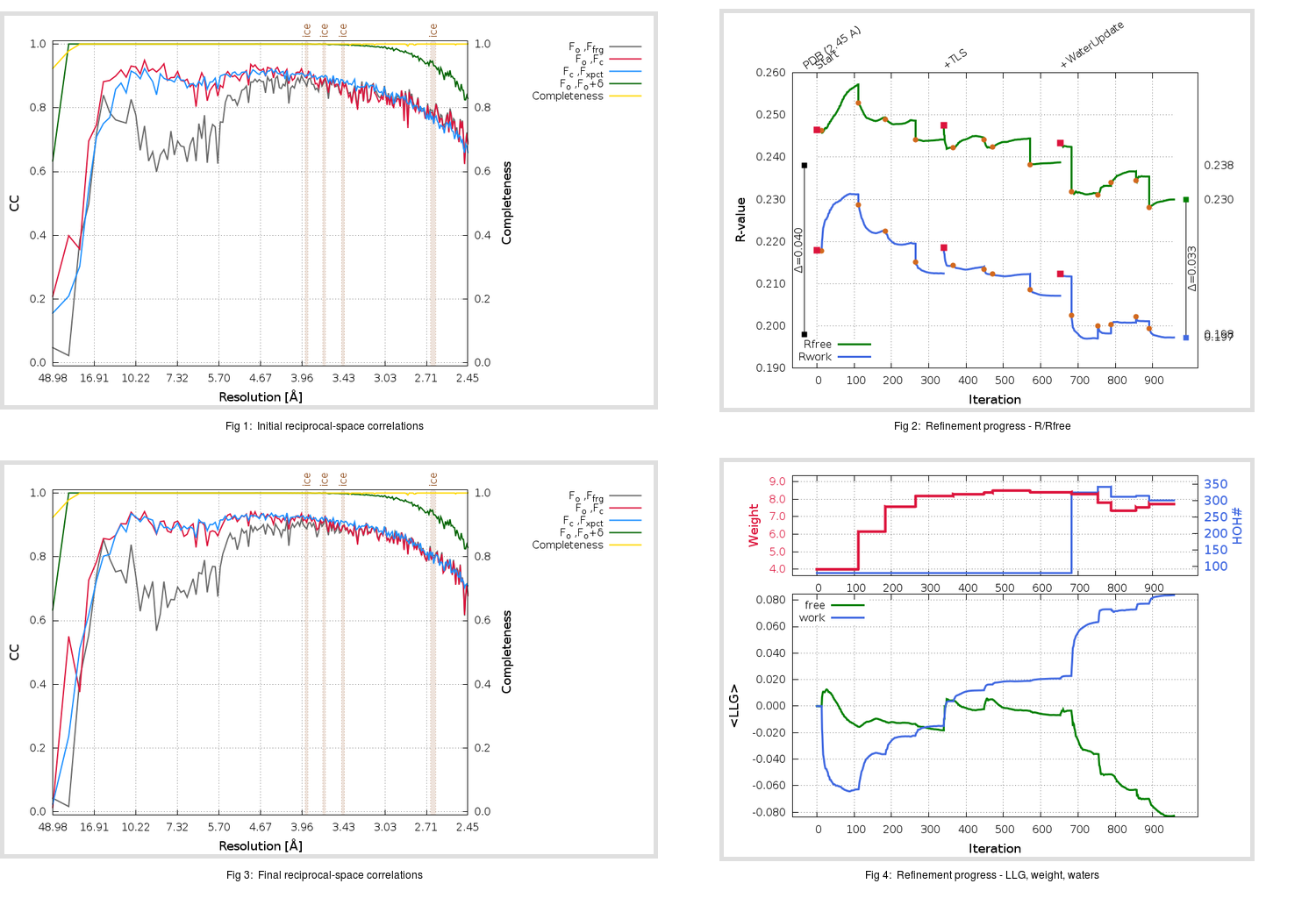Content:

```    Diffraction limits & principal axes of ellipsoid fitted to diffraction cut-off surface:
2.441         1.0000   0.0000   0.0000       a*
2.441         0.0000   1.0000   0.0000       b*
2.413         0.0000   0.0000   1.0000       c*
```

## Deposited

` `
 Date deposited Date data collection Resolution R, Rfree 20200221 20200217 2.45 0.1960 0.2380

Molprobity (CCP4 7.0 version) summary:

```Ramachandran outliers =   0.00 %
favored =  96.44 %
Rotamer outliers      =   4.60 %
C-beta deviations     =     0
Clashscore            =   5.07
RMS(bonds)            =   0.0084
RMS(angles)           =   1.21
MolProbity score      =   2.01
Resolution            =   2.45
R-work                =   0.1960
R-free                =   0.2380
```

```Number of waters      =    80

<B> (all atoms) =   64.83 ( sd =   16.96 ) for       6556 non-hydrogen atoms
<B>   (protein) =   64.68 ( sd =   16.90 ) for       6419 non-hydrogen atoms
<B>     (water) =   58.07 ( sd =    6.86 ) for         80 non-hydrogen atoms
<B>    (others) =   91.40 ( sd =    7.54 ) for         57 non-hydrogen atoms

B min/max       (all non-hydrogen atoms) =   37.51 /  150.60
B min/max   (protein non-hydrogen atoms) =   37.51 /  150.60
B min/max     (water non-hydrogen atoms) =   45.48 /   75.97
B min/max     (other non-hydrogen atoms) =   77.33 /  111.98
```

## BUSTER (re-)refinement

` `

Molprobity (CCP4 7.0 version) summary:

```Ramachandran outliers =   0.13 %
favored =  97.08 %
Rotamer outliers      =   5.03 %
C-beta deviations     =     3
Clashscore            =   2.69
RMS(bonds)            =   0.0123
RMS(angles)           =   1.53
MolProbity score      =   1.75
Resolution            =   2.45
R-work                =   0.1972
R-free                =   0.2300
```

```Number of waters      =   300

<B> (all atoms) =   69.94 ( sd =   18.53 ) for       6776 non-hydrogen atoms
<B>   (protein) =   69.66 ( sd =   18.64 ) for       6419 non-hydrogen atoms
<B>     (water) =   70.32 ( sd =   12.04 ) for        300 non-hydrogen atoms
<B>    (others) =   99.60 ( sd =    2.36 ) for         57 non-hydrogen atoms

B min/max       (all non-hydrogen atoms) =   30.36 /  154.91
B min/max   (protein non-hydrogen atoms) =   42.14 /  154.91
B min/max     (water non-hydrogen atoms) =   30.36 /  117.24
B min/max     (other non-hydrogen atoms) =   93.57 /  103.42
```

Refinement progression:Results:

` `
 File Remark 6M0J_aB_refine.01_03_refine.pdb.gz exact refinement commands are in header 6M0J_aB_refine.01_03_refine.mtz.gz including original deposited data and several re-refinement map coefficients 6M0J_aB_refine.01_03_BUSTER_model.cif.gz including any non-standard compound restraints 6M0J_aB_refine.01_03_BUSTER_refln.cif.gz